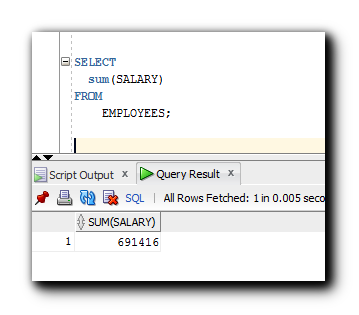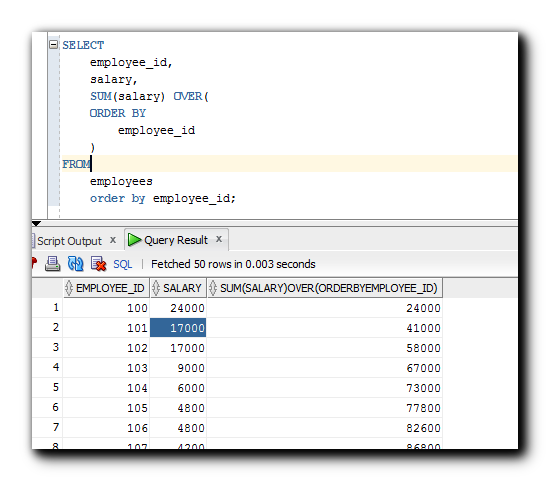# Analytic Functions in Oracle

An introductory discussion about Oracle Analytic Functions which are used to calculate or compute Aggregate values, based on a group of rows.

Analytic functions are used to calculate or compute aggregate values based on a group of rows, it is also called as a Window. This determines the range of rows used to perform the calculations from the current row. The analytic functions only work on top of the results that are retrieved from the SQL queries. This helps us to analyze the data much easier.

These types of functions also help us in calculating cumulative totals. The most common use of these functions on identifying the ranks for example if we need to know the top persons who are highly paid or top 5 sales executive those who have done the best sales for a year.Their functions are not affected by “Group by” or “Where” or “Having” clause in Oracle.

Main differences between analytic and aggregate functions

The main difference between these two functions is that aggregate functions are used to return a single result value based on a group of rows. For example, if we need to identify the total number of audits till date from Audit table, then we need to use count function to determine the number of row in audit object. Now if we use the analytic way then this will return multiple rows with certain results within a window.

Examples below show how to retrieve the results from an audit table using analytic function and on aggregate function.

Below is the select clause using aggregate function “sum” with expression list i.e. Salary column from Employee table joining with from clause returns the total amount spent by a company on salary.Now let us see if we can use analytical functions on the same table to get different results.From the above SQL, we can see that the same Sum functions are now used as analytic functions to get the sum, but on cumulative salary. When we use the analytic function, we must use “OVER” and we also need to use order by or partition by as analytic.